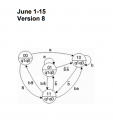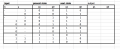# Deriving a state table for a finite state machine

#### dinkofelic23

Joined Nov 28, 2020
43
Hi, I am currently trying to solve this:The assignment is to create a state table of the finite state machine depicted above.
This is how far I get:At almost all examples I have seen online, the output is shown below the state, for example instead of q1q0 it usually says the output. But here it is different. My question is, how do I read out the output? Is the output the next state?

#### Delta Prime

Joined Nov 15, 2019
1,197
Monkey see monkey do. Use your imagination make it your own. It'll come to you.

#### Papabravo

Joined Feb 24, 2006
17,318
Hint: There are two type of finite state machines, called Moore and Mealy. What is the difference? Why is that important for this problem?

#### WBahn

Joined Mar 31, 2012
26,398
The diagram does not appear to define what the output is in each state. If the point of the assignment is to just come up with the state transition table, then the output may simply not be relevant to what the goal of the assignment is. In that case, you aren't designing a complete system, just working a specific part of the problem.

#### dinkofelic23

Joined Nov 28, 2020
43
The diagram does not appear to define what the output is in each state. If the point of the assignment is to just come up with the state transition table, then the output may simply not be relevant to what the goal of the assignment is. In that case, you aren't designing a complete system, just working a specific part of the problem.
Then I am not crazy for not understanding. The point of the assignment is to make the state table and from there make K-maps and derive a boolean function in minimized form to then make an AND-OR network and lastly to build the state machine using D Flip-flops and other gates.

But in order to make the K-map I need to have outputs to get min and maxterms... So therefore I turned here for advice, because I have searched all day with no success.

#### Papabravo

Joined Feb 24, 2006
17,318
Then I am not crazy for not understanding. The point of the assignment is to make the state table and from there make K-maps and derive a boolean function in minimized form to then make an AND-OR network and lastly to build the state machine using D Flip-flops and other gates.

But in order to make the K-map I need to have outputs to get min and maxterms... So therefore I turned here for advice, because I have searched all day with no success.
Not exactly. If I told you that one type of machine has the property that the outputs are ONLY a function of the state, what is the simplest possible function that you can imagine?

#### dinkofelic23

Joined Nov 28, 2020
43
Not exactly. If I told you that one type of machine has the property that the outputs are ONLY a function of the state, what is the simplest possible function that you can imagine?
Moore machine? Since the output is delfined by only the present state?
Does that mean that the output is the same as the state it is in? So for example 01 has the output 01?

#### Papabravo

Joined Feb 24, 2006
17,318
Moore machine? Since the output is delfined by only the present state?
Does that mean that the output is the same as the state it is in? So for example 01 has the output 01?
I think that interpretation would pass muster under the present circumstances. Some might consider it r/malicious compliance, but what else are you gonna' do.

#### WBahn

Joined Mar 31, 2012
26,398
Then I am not crazy for not understanding. The point of the assignment is to make the state table and from there make K-maps and derive a boolean function in minimized form to then make an AND-OR network and lastly to build the state machine using D Flip-flops and other gates.

But in order to make the K-map I need to have outputs to get min and maxterms... So therefore I turned here for advice, because I have searched all day with no success.
Your K-maps would be for the state transition excitation logic.

•Papabravo

#### WBahn

Joined Mar 31, 2012
26,398
Moore machine? Since the output is delfined by only the present state?
Does that mean that the output is the same as the state it is in? So for example 01 has the output 01?
The output for each state is simply not defined on the state diagram. If you want to pretend that the output is the state variables, you can. At least that is trivially easy to do.

#### Analog Ground

Joined Apr 24, 2019
456
From that state diagram, I infer the state variable is the same as the outputs. This works because each state has different outputs. So, it is a Moore machine. You state table is a good start but you should put it in a more standard form. By the way, there are some errors and you should not progress to the k-maps or logic equations until getting the state table to match the diagram. Here is an example of a form which will serve you well in this problem.

https://www.chegg.com/homework-help...-b-c-d-four-rows-table-give-new-stat-q4721580

Put the "present states" in the left column. Then, to the right a column for each combinations of the two inputs. So, there will be 4 "input columns". Each row entry for these input columns will be the next state for the combination of the present state and the input combination. You won't need the "Output" column since your outputs are just the state info. Then, build you K-maps from there. With this form, the k-maps practically build themselves.

If you want to give the states names, go ahead. Not necessary but it can help keep things straight. Like A, B, C. D or whatever suits you.

Here is an example of a state table of the form I am suggesting with two inputs. Notice how this person has filled the table with state names. Eventually the names are replaced with the output values in your state diagram. You can go either way to start.

https://www.chegg.com/homework-help...state-table-shown-find-equivalent-s-q17378659

Last edited:

#### dinkofelic23

Joined Nov 28, 2020
43
Or perhaps I should change it so that under next state, where it says q1q0 = 00 it should be ba = 00 since that is the input, then in the K-map one side should be q1q0 as the state and the other side ba as the input? I am working with them right, I'll post soon

#### dinkofelic23

Joined Nov 28, 2020
43

#### Analog Ground

Joined Apr 24, 2019
456
This looks great (post #14)! Now do you know how to build the four input K-maps? How many k-maps are there? What are the outputs of the K-maps? Remember, now is when you need to decide on what type of flip-flop you will use.

Last edited:

#### dinkofelic23

Joined Nov 28, 2020
43

#### Analog Ground

Joined Apr 24, 2019
456
View attachment 224354
Thanks! This is what I managed to scrap together. One K-map for the next state of q1 (q1+) and another for the next state of q0 (q0+)

In the instructions of the assignment they hinted at making K-maps this way:
View attachment 224355
I am not sure what is meant by the left K-map of the hints. Maybe the format is condensing two K-maps into one. With one output on the left of the vertical bar in a cell and the other output to the right of the vertical bar in a cell. Personally, I would never do it this way. Too confusing. I would do two maps like the other two. Also, the q+ format is not familiar. The main thing is to realize the output of the k-maps are the inputs to the flip-flops. Perhaps this is what is meant by q+. For example, JKFF has a J and a K input, a DFF has a D input, etc. So, if I ignore the left k-map of the hints, and assume q+ means the input to a DFF. Then, the hint is very, very helpful. Now, you should see how easy it is to fill in the k-maps from the state table.

EDit: I posted just when you did. Let me look at your k-maps.